# Examples

The GitHub repository contains a number of standard examples on how to run the code. The examples for both magnetostatic calculations and micromagnetic simulations can be found in matlab/examples in the MagTense repository. Here we present the results from some of these examples

Magnetostatic examples:

In example 001 the magnetic field generated by a 5 cm x 5 cm x 5 cm square permanent magnet cube with a remanence of 1.2 T, an easy axis relative permeability of 1.06 and an off-axis relative permeability of 1.17 is computed. The direction of the remanence is parallel to four of the sides of the cube. The computed magnetic field is shown in the image below. Notice how the field opposes the magnetization inside the cube.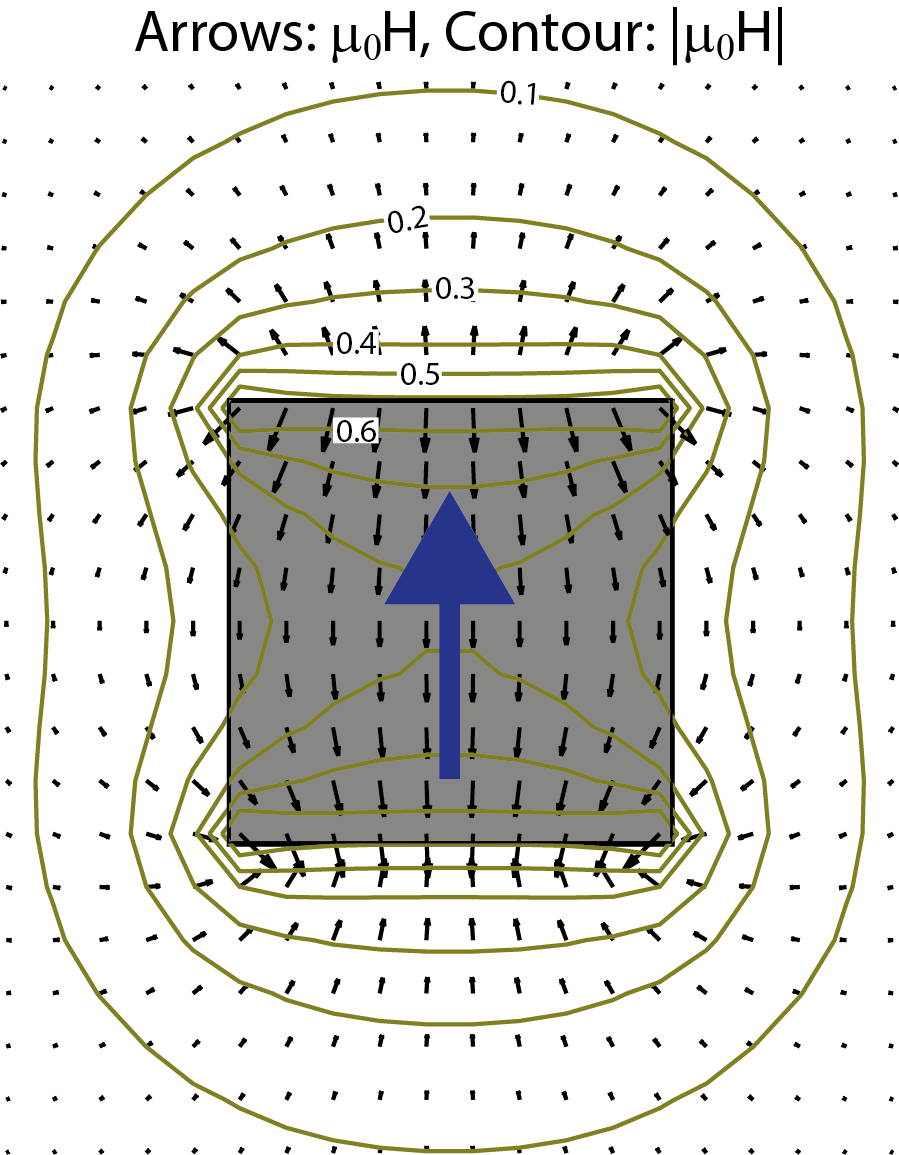Micromagnetic examples:

MagTense has the µmag standard problems 2, 3 and 4 as examples with fulling working example code for each of these. The detailed physics and results are presented in a scientific papers as well [PublisherarXiv].

µmag standard problems 2

In standard problem 2 the coercivity of a slab of magnetic material is calculated under a reversing applied field. The coercivity as calculated using the MagTense framework as function of the dimensions of the slab normalized by the exchange length is shown below, along with tabulated solutions available from the &#181;mag homepage.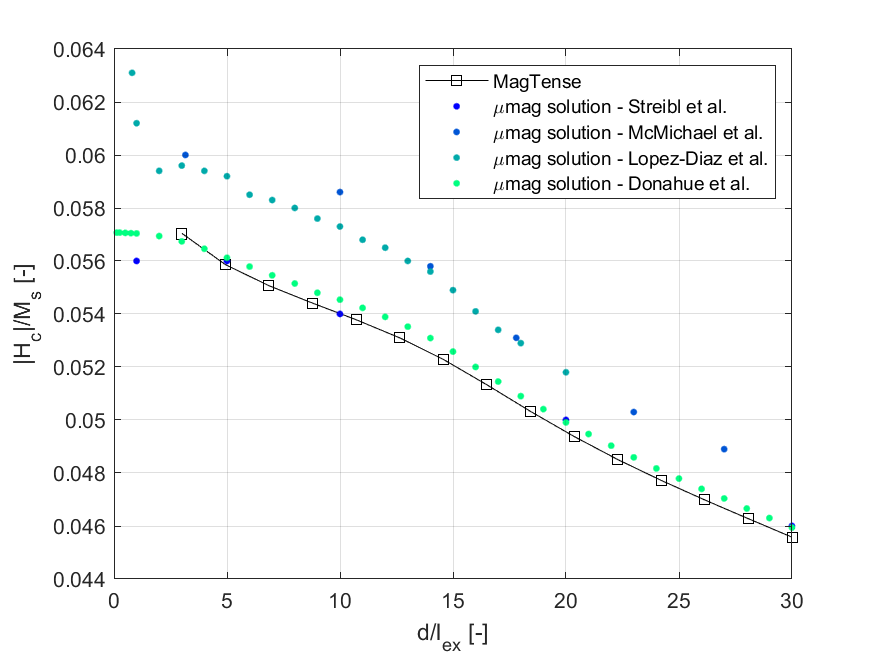µmag standard problems 3

In standard problem 3 the lowest energy state of a cube is calculated. When the dimensions of the cube is changed, the lowest energy state changes from a flower state to a vortex state. The standard problem requires a computation of the dimensions of the cube at which the cross-over of the energy states happen. Below this dimension given as a function of the resolution of uniform grid used to mesh the cube along with tabulated solutions from the µmag homepage.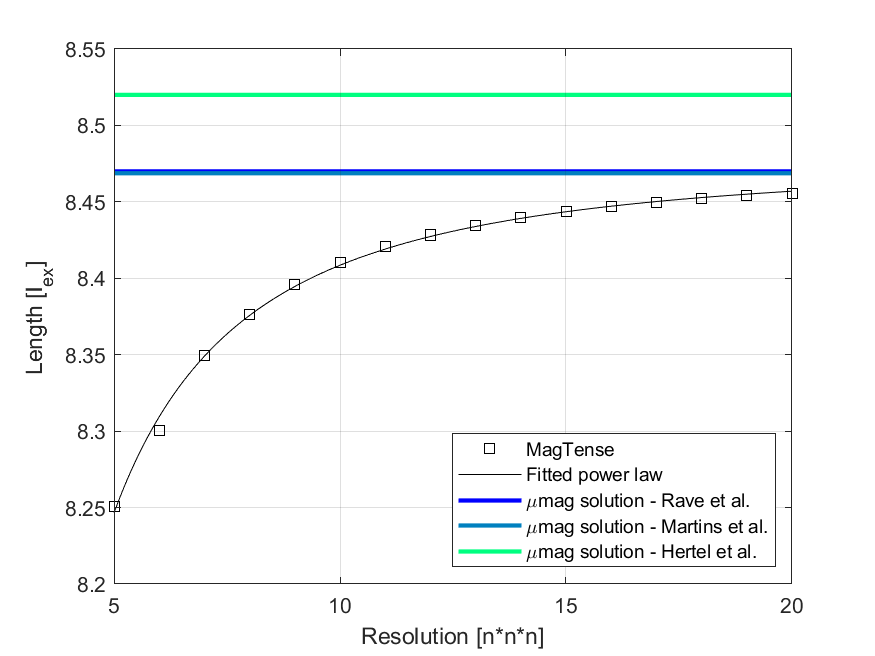µmag standard problems 4

In standard problem 4 the time evolution of a slab of magnetic material in a magnetic field energy state of a cube is calculated. Below we show the time evolution of the three components of the magnetization for the slab along with tabulated solutions from the µmag homepage.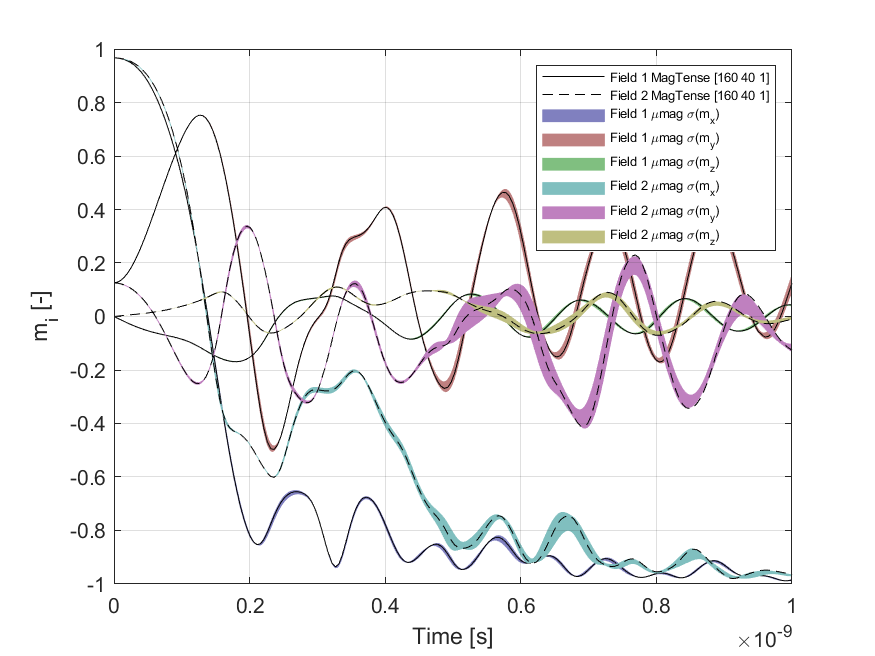Below is shown an animation of the magnetization as the field is changed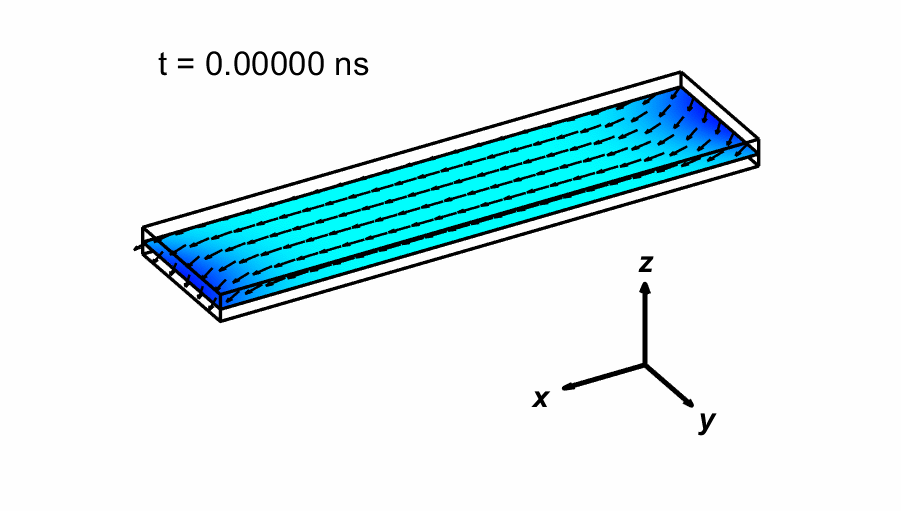https://www.magtense.org/examples
3 JULY 2022